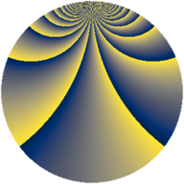# Properties

 Label 1610.2.bcLevel $1610$ Weight $2$ Character orbit 1610.bc Rep. character $\chi_{1610}(29,\cdot)$ Character field $\Q(\zeta_{22})$ Dimension $720$ Sturm bound $576$

# Related objects

## Defining parameters

 Level: $$N$$ $$=$$ $$1610 = 2 \cdot 5 \cdot 7 \cdot 23$$ Weight: $$k$$ $$=$$ $$2$$ Character orbit: $$[\chi]$$ $$=$$ 1610.bc (of order $$22$$ and degree $$10$$) Character conductor: $$\operatorname{cond}(\chi)$$ $$=$$ $$115$$ Character field: $$\Q(\zeta_{22})$$ Sturm bound: $$576$$

## Dimensions

The following table gives the dimensions of various subspaces of $$M_{2}(1610, [\chi])$$.

Total New Old
Modular forms 2960 720 2240
Cusp forms 2800 720 2080
Eisenstein series 160 0 160

## Trace form

 $$720q + 72q^{4} - 8q^{5} + 88q^{9} + O(q^{10})$$ $$720q + 72q^{4} - 8q^{5} + 88q^{9} - 8q^{11} + 8q^{14} + 20q^{15} - 72q^{16} + 32q^{19} + 8q^{20} - 8q^{21} + 52q^{25} + 16q^{29} + 44q^{30} - 32q^{31} - 16q^{34} - 8q^{35} - 88q^{36} + 56q^{39} + 32q^{41} + 8q^{44} + 8q^{45} + 72q^{49} + 16q^{51} + 120q^{55} - 8q^{56} + 144q^{59} + 68q^{60} + 16q^{61} + 72q^{64} - 128q^{66} + 392q^{69} + 8q^{70} - 360q^{71} + 184q^{74} - 116q^{75} - 32q^{76} + 48q^{79} + 36q^{80} - 80q^{81} + 8q^{84} + 40q^{85} - 40q^{86} - 96q^{89} + 80q^{91} - 16q^{94} + 20q^{95} + 24q^{99} + O(q^{100})$$

## Decomposition of $$S_{2}^{\mathrm{new}}(1610, [\chi])$$ into newform subspaces

The newforms in this space have not yet been added to the LMFDB.

## Decomposition of $$S_{2}^{\mathrm{old}}(1610, [\chi])$$ into lower level spaces

$$S_{2}^{\mathrm{old}}(1610, [\chi]) \cong$$ $$S_{2}^{\mathrm{new}}(115, [\chi])$$$$^{\oplus 4}$$$$\oplus$$$$S_{2}^{\mathrm{new}}(230, [\chi])$$$$^{\oplus 2}$$$$\oplus$$$$S_{2}^{\mathrm{new}}(805, [\chi])$$$$^{\oplus 2}$$